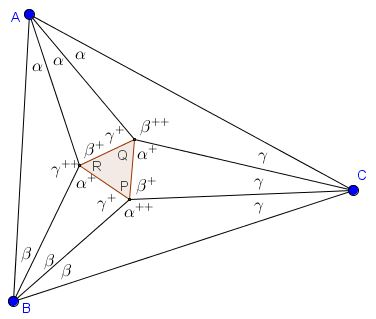# Morley's Miracle, Bella Bollobás' Trigonometric Proof

The three points of intersection of the adjacent trisectors of the angles of any triangle form an equilateral triangle.For simplicity, let us write $x^{+}$ for $x+\pi/3.$ We claim that the angles of the six triangles are as in the diagram below:Note that the assignment of angles is consistent with the requirement that the sum of angles in a triangle is $\pi.$ Simple calculations show that this is indeed so and that the angles in $\Delta PQR$ are each $60^{\circ}.$ We do know that the magnitudes of the angles $AQC,$ $BPC,$ and $ARB$ are as shown. To establish Morley's theorem we need to verify that other angles (those with a single $^{+})$ are distributed as in the diagram.

We assume that the circumradius of $\Delta ABC$ equals $1/2.$ This makes $BC=\sin 3\alpha,$ $AC=\sin 3\beta,$ and $AB=\sin 3\gamma.$ With the Law of Sines, we have, in $\Delta ABR,$ $\displaystyle AR=\frac{\sin\beta}{\sin\gamma^{++}}\sin 3\gamma$ and, in $\Delta ACQ,$ $\displaystyle AQ=\frac{\sin\gamma}{\sin\beta^{++}}\sin 3\beta.$

Hence, in order that in $\Delta ARQ,$ the angles be as claimed, implying, in particular, $\displaystyle\frac{AR}{AQ}=\frac{\sin\gamma^{+}}{\sin\beta^{+}},$ we need

$\sin 3\beta\sin\gamma\sin\gamma^{+}\sin\gamma^{++}=\sin 3\gamma\sin\beta\sin\beta^{+}\sin\beta^{++}.$

But this is indeed so because

$\sin 3x=4\sin x \sin x^{+} \sin x^{++}=3\sin x-4\sin^{3}x.$

### References

1. B. Bollobás, The Art of Mathematics, Cambridge University Press, 2006, p. 126-127### Morley's Miracle

#### Invalid proofs• Equilateral and 3-4-5 Triangles
• Rusty Compass Construction of Equilateral Triangle
• Equilateral Triangle on Parallel Lines
• Equilateral Triangle on Parallel Lines II
• When a Triangle is Equilateral?
• Viviani's Theorem
• Viviani's Theorem (PWW)
• Tony Foster's Proof of Viviani's Theorem
• Viviani in Isosceles Triangle
• Viviani by Vectors
• Slanted Viviani
• Slanted Viviani, PWW
• Triangle Classification
• Napoleon's Theorem
• Sum of Squares in Equilateral Triangle
• A Property of Equiangular Polygons
• Fixed Point in Isosceles and Equilateral Triangles
• Parallels through the Vertices of Equilateral Triangle
•